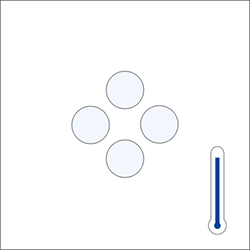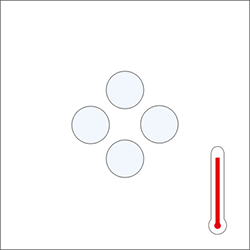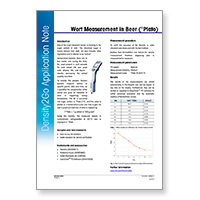# What is Density?

## All You Need to Know about Density Measurement### What is Density? - How to measure Density? – What are the main applications of Density?

In this video, you will become an expert on how to measure density of liquids and the best methods to measure it.HydrometersMeasuring principle

• Glass body inserted into sample
• Glass body floats at a certain level due to the buoyancy and mass of the hydrometer, dependent on sample density
• Level of equilibration shows the density on the calibrated scale

Strengths

• Simple, inexpensive
• Used for a quick check of an approximate density value

Weaknesses

• User-dependent results
• Takes a long time to equilibrate the temperature
• Small measuring range (typically takes 20 hydrometers to cover a wide range)
• Large sample volume required (140 mL to 600 mL)
• Difficult to clean
• Not suitable for GLP
• Breakable
• Sample must be removed from sample container and poured into the hydrometer

PycnometersPycnometers

Measuring principle

• Glass beaker of defined volume
• Weighed without sample (M1), then with a sample (M2)
• Density calculated based on the following formula:
Density = (M2 − M1)/Flask Volume

Strengths

• Inexpensive
• Directly related to the definition of density (mass divided by volume): ideal for academia / education

Weaknesses

• User-dependent results
• Pycnometers are calibrated for a certain temperature, e.g. 20 °C, so measurements are only valid at that temperature! The sample must be equilibrated to the calibration temperature.
• Density must be calculated
• Typical sample volume required is 25 mL
• High level of user training required to ensure accurate, trustworthy measurements
• Sample must be removed from sample container and added to pycnometer

Digital Density MetersDigital Density Meters

Measuring principle

• Oscillating U-tube
• A hollow glass tube vibrates at a certain frequency. This frequency changes when the tube is filled with the sample: the higher the mass of the sample, the lower the frequency measurement is and converted into density. A built-in Peltier thermostat controls the temperature precisely of the benchtops instrument (no water bath required)

Strengths

• Easy to use
• Small sample volume
• Automatic measurement means results are operator independent
• Built-in temperature compensation
• Offers storage of up to 1100 results and the possibility to connect to PC software for data management
• Sample can be measured directly from the sample container

Weaknesses

• More expensive in comparison to hydrometers or pycnometers

### Manual to Digital Density MeasurementMolecule at a given temperature
(slight movements)Same molecule when temperature increases
(moving further apart)Mass in vacuum = True density

To illustrate the difference between true density and apparent density, we have a pycnometer placed onto a balance. When it is filled with a liquid, it weighs less in air than in a vacuum due to the buoyancy effect of air.

Many official density tables are still based on apparent density. Digital density meters deliver results in different units and concentrations, check out their specifications.Mass in air = Apparent density## What Is the Difference between Density and Mass?

Mass is a measure of how much matter there is within an object or liquid while density expresses how much mass there is per a certain amount of volume.

For example, 10 kg of steel and 10 kg of feathers have the same mass, but different volumes therefore they have different densities.

## Why Can Density Be Used to Identify a Sample?

Density can easily be used to identify a pure sample because each element has a unique density. After a measurement, the density of the sample in question can be looked up to see what it corresponds to.

## How Is the Density of Solutions Measured?

Let's take a solution of ethanol in water as an example.

As shown before, at 20 °C pure water has a density of d = 0.9982 g/cm3, and pure ethanol has a density of d = 0.7892 g/cm3 at 20 °C. A solution of ethanol/water will have a density value which depends on the concentration of the solution.

## Is Density Directly Proportional to Pressure?

Density is directly proportional to the local air pressure but indirectly proportional to temperature. At a constant temperature, when pressure increases density increases. Learn more about the relationship between density of liquids and pressure here.

## What Are Some Typical Applications of Density Measurement?Some applications of density measurement includes the determination of alcohol concentration in spirits, control of fermentation process in wine and beer production, Brix (sugar content) measurement of intermediate and final products in food and beverages products. Density and other concentration as API gravity in heavy oils, paraffin and lubricants in the Petrochemical segment. Density (specific gravity) in battery acid in the automobile industry, as well as other solvents, acids and bases in the chemical industry. Lastly, there are many applications in the pharmaceutical industry, such as the density measurement of specific gravity in cosmetics, personal care products, and many more. Click on the links above to read our detailed applications or access our expertise library to find the right application note according to your sample.

## What Is the Density of Air?

The density of air is 0.00120 g/cm3 at 20°C and under atmospheric pressure of 101.325 kPa (i.e. at sea level). This atmospheric pressure changes with the weather conditions (lower pressure when rainy or snowy) and with the altitude (lower pressure at high altitude than at sea level). At an elevation of 440 m above sea level, for example, the atmospheric pressure (yearly mean) is 96.12 kPa only and the mean air density becomes 0.00114 g/cm3 at 20°C.

## What Is the Density of Water?

The density of water is 0.99820 g/cm3 at 20°. The density of water changes with the temperature. It increases from 0°C to 4°C (where it is nearly 1) and then decreases from 4°C to higher temperatures.

## What Is the Influence of Viscosity on Digital Density Measurement?

Sample viscosity has an impact on the density measured with a digital density meter. The higher shear force which occurs between the fluid and the tube wall results in slowing down the oscillation frequency, thus showing a higher density value. Modern digital density meters therefore have a built-in viscosity correction, which compensates for this effect to show correct results.